# 『 论文阅读』LightGBM原理-LightGBM: A Highly Efficient Gradient Boosting Decision Tree

17年8月LightGBM就开源了，那时候就开始尝试上手，不过更多还是在调参层面，在作者12月论文发表之后看了却一直没有总结，这几天想着一定要翻译下，自己也梳理下GBDT相关的算法。

# Abstract

Gradient Boosting Decision Tree (GBDT)非常流行却鲜有实现，只有像XGBoost和pGBRT。当特征维度较高和数据量巨大的时候，其实现中仍然存在效率和可扩展性的问题。一个主要原因就是对于每一个特征的每一个分裂点，都需要遍历全部数据计算信息增益，这一过程非常耗时。针对这一问题，本文提出两种新方法：Gradient-based One-Side Sampling (GOSS) 和Exclusive Feature Bundling (EFB)基于梯度的one-side采样互斥的特征捆绑）。在GOSS中，我们排除了重要的比例-具有小梯度的实例，只用剩下的来估计信息增益，我们证明，这些梯度大的实例在计算信息增益中扮演重要角色，GOSS可以用更小的数据量对信息增益进行相当准确的估计（疑问：怎么证明，数学 or 实验？）。对于EFB，我们捆绑互斥的特征（什么是互斥特征：例如特征间很少同时非零。），来降低特征的个数。我们证明完美地捆绑互斥特征是NP难的，但贪心算法能够实现相当好的逼近率，因此我们能够在不损害分割点准确率许多，有效减少特征的数量。（牺牲一点分割准确率降低特征数量），这一算法命名为LightGBM。在多个公共数据集实验证明，LightGBM加速了传统GBDT训练过程20倍以上，同时达到了几乎相同的精度。

# 1. Introduction

GBDT因为其的有效性、准确性、可解释性，成为了广泛使用的机器学习算法。GBDT在许多机器学习任务上取得了最好的效果（ state-of-the-art），例如多分类，点击预测，排序。但最近几年随着大数据的爆发（特征量和数据量），GBDT面临平衡准确率和效率的调整。

GBDT缺点：对于每一个特征的每一个分裂点，都需要遍历全部数据来计算信息增益。因此，其计算复杂度将受到特征数量和数据量双重影响，造成处理大数据时十分耗时。

Gradient-based One-Side Sampling (GOSS)：GBDT虽然没有数据权重，但每个数据实例有不同的梯度，根据计算信息增益的定义，梯度大的实例对信息增益有更大的影响，因此在下采样时，我们应该尽量保留梯度大的样本（预先设定阈值，或者最高百分位间），随机去掉梯度小的样本。我们证明此措施在相同的采样率下比随机采样获得更准确的结果，尤其是在信息增益范围较大时。

Exclusive Feature Bundling (EFB)：通常真是应用中，虽然特征量比较多，但是由于特征空间十分稀疏，是否可以设计一种无损的方法来减少有效特征呢？特别在稀疏特征空间上，许多特征几乎是互斥的（例如许多特征不会同时为非零值，像one-hot），我们可以捆绑互斥的特征。最后，我们将捆绑问题归约到图着色问题，通过贪心算法求得近似解。

# 2. Preliminaries

## 2.1 GBDT and Its Complexity Analysis

GBDT是一种集成模型的决策树，顺序训练决策树。每次迭代中，GBDT通过拟合负梯度（残差）来学到决策树。

histogram-based算法通过直方图寻找最优切分点，其建直方图消耗O(#data * #feature)，寻找最优切分点消耗O(#bin * # feature)，而#bin的数量远小于#data，所以建直方图为主要时间消耗。如果能够减少数据量或特征量，那么还能够够加速GBDT的训练。

## 2.2 Related Work

GBDT有许多实现，如XGBoost，PGBRT，Scikit-learn，gbm in R。Scikit-learn和gbm in R实现都用了预排序，pGBRT使用了直方图算法那，XGBoost支持预排序和直方图算法，由于XGBoost胜过其他算法，我们用它作为baseline。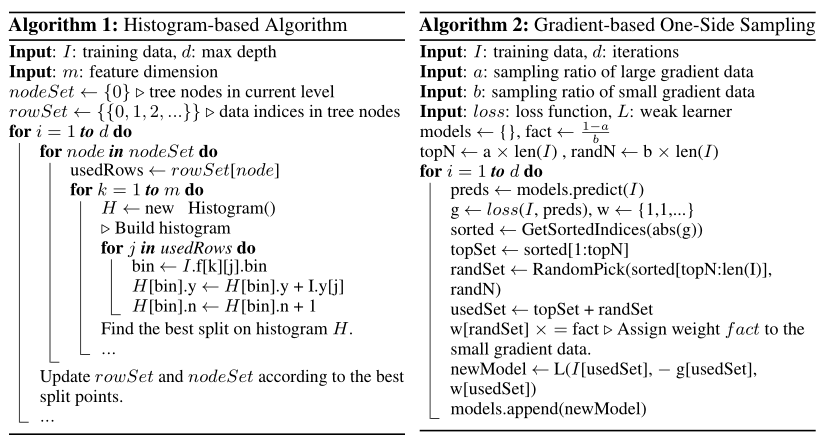# 3. Gradient-based One-Side Sampling

GOSS是一种在减少数据量和保证精度上平衡的算法。

## 3.1 Algorithm Description

GOSS保留所有的梯度较大的实例，在梯度小的实例上使用随机采样。为了抵消对数据分布的影响，计算信息增益的时候，GOSS对小梯度的数据引入常量乘数。GOSS首先根据数据的梯度绝对值排序，选取top a个实例。然后在剩余的数据中随机采样b个实例。接着计算信息增益时为采样出的小梯度数据乘以(1-a)/b，这样算法就会更关注训练不足的实例，而不会过多改变原数据集的分布。

## 3.2 Theoretical Analysis

GBDT使用决策树，来学习获得一个将输入空间映射到梯度空间的函数。假设训练集有n个实例${x_1,...,x_n}$，特征维度为s。每次梯度迭时，模型数据变量的损失函数的负梯度方向表示为$g_1,...,g_n$，决策树通过最优切分点（最大信息增益点）将数据分到各个节点。GBDT通过分割后的方差衡量信息增益。

$V_{j|O}(d) = \frac{1}{n_O} ( \frac{ ( \sum_{ \{x_i \in O: x_{ij} \le d \} } g_i )^2 }{n^j_{l|O}(d)} + \frac{ ( \sum_{ \{x_i \in O: x_{ij} > d \} } g_i )^2 }{n^j_{r|O}(d)} )$

1. 首先根据数据的梯度将训练降序排序。

2. 保留top a个数据实例，作为数据子集A。

3. 对于剩下的数据的实例，随机采样获得大小为b的数据子集B。

4. 最后我们通过以下方程估计信息增益:

$\tilde{V}_j (d) = \frac{1}{n} ( \frac{ ( \sum_{ {x_i \in A: x_{ij} \le d } } g_i + \frac{1-a}{b}\sum_{ {x_i \in B: x_{ij} \le d } } g_i )^2 }{n^j{l}(d)} + \frac{ ( \sum_{ {x_i \in A: x{ij} > d } } g_i + \frac{1-a}{b}\sum_{ {x_i \in B: x{ij} > d } } g_i )^2 }{n^j_{r}(d)} ) \tag{1}$

Theorem 3.2：我们定义GOSS近似误差为$\varepsilon(d) = |\tilde{V}_j(d) - V_j(d) |$$\bar{g}_l^j(d)=\frac{\sum_{x_i \in (A \cup A^c)_l} |g_i|}{n_l^j(d)}$，$\bar{g}l^j(d)=\frac{\sum{x_i \in (A \cup A^c)_r} |g_i|}{n_r^j(d)}$，概率至少是$1- \delta$，有：
$\varepsilon(d) \le C_{a, b}^2 \ln (1/\delta) * \max\{ \frac{1}{n_l^j(d)} , \frac{1}{n_r^j(d)} \} + 2*D*C_{a,b} \sqrt{ \frac{\ln(1/\delta)}{n}} \tag{2}$

1. GOSS的渐近逼近比率$O(\frac{1}{n_l^j(d)}+ \frac{1}{n_r^j(d)} + \frac{1}{\sqrt{n}}) $。如果数据分割不是极不平衡（例如$n_l^j(d) \ge O(\sqrt{n}) $和$ n_r^j \ge O(\sqrt{n})$），那么不等式(2)中近似误差将由第二项主导，当n趋于无穷（数据量很大）时$O(\sqrt{n})$将趋于0，即数据量越大，误差越小，精度越高。
2. 随机采样是GOSS在a=0的一种情况。多数情况下，GOSS性能优于随机采样，即以下情况：$C_{0, \beta} > C_{a, \beta-a}$，即$\frac{\alpha_a}{\sqrt{\beta}} > \frac{1-a}{\sqrt{\beta-a}}$，其中$\alpha_a = \max_{x_i \in A \cup A^c} |g_i| / \max_{x_i \in A^c} |g_i|$

# 4 Exclusive Feature Bundling

1. 怎么判定那些特征应该绑在一起（build bundled）？

2. 怎么把特征绑为一个（merge feature）？

## 4.1 bundle（什么样的特征被绑定）？

**理论 4.1：**将特征分割为较小量的互斥特征群是NP难的。

**算法3：**基于上面的讨论，我们设计了算法3，伪代码见下图，具体算法：

1. 建立一个图，每个点代表特征，每个边有权重，其权重和特征之间总体冲突相关。
2. 按照降序排列图中的度数来排序特征。
3. 检查排序之后的每个特征，对他进行特征绑定或者建立新的绑定使得操作之后的总体冲突最小。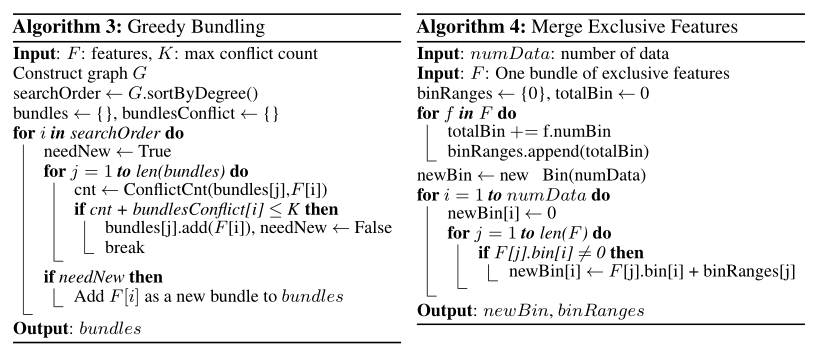## 4.2 merging features(特征合并)

EFB算法能够将许多互斥的特征变为低维稠密的特征，就能够有效的避免不必要0值特征的计算。实际，通过用表记录数据中的非零值，来忽略零值特征，达到优化基础的直方图算法。通过扫描表中的数据，建直方图的时间复杂度将从O(#data)降到O(#non_zero_data)。当然，这种方法在构建树过程中需要而额外的内存和计算开销来维持预特征表。我们在lightGBM中将此优化作为基本函数，因为当bundles是稀疏的时候，这个优化与EFB不冲突（可以用于EFB）。

# Experiments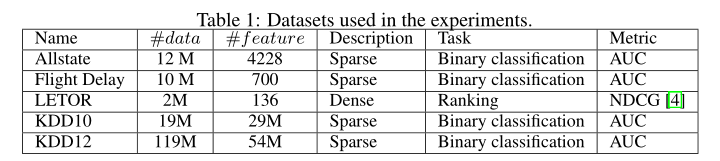Allstate是保险和航空延误数据都包含了大量的one-hot特征。

## 5.1 Overall Comparison

XGBoost和lightGBM without GOSS 和EFB（lgb baseline），作为比较的基准。XGBoost使用了两个版本：xgb_exa(预排序)和xgb_his(直方图算法)。对于xgb_exa做了参数调整，使XGBoost长成和其他算法相似的树。并且调整参数在速度和准确率间平衡。对于Allstate、KDD10 和 KDD2012，设置a = 0.05 , b = 0.05 ，对于航空延误和LETOR，我们设置a = 0.1 , b = 0.1 ，数据集EFB我们设置γ = 0 。所有的算法有固定迭代次数。在一定迭代次数内，我们取最高的分数。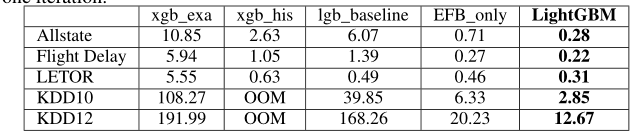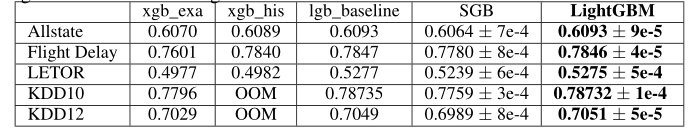## 5.2 Analysis on GOSS

**速度上：**GOSS具有加速训练的能力，表2中可以看出GOSS几乎加速了两倍。虽然GOSS用了10%-20%的数据训练，但是由于有一些额外的计算，所以加速并不和数据量成反比，但是GOSS仍然极大加速了训练。

**精度上：**从表4中可以看出，同样采样率，GOSS精度总比SGB好。

# References